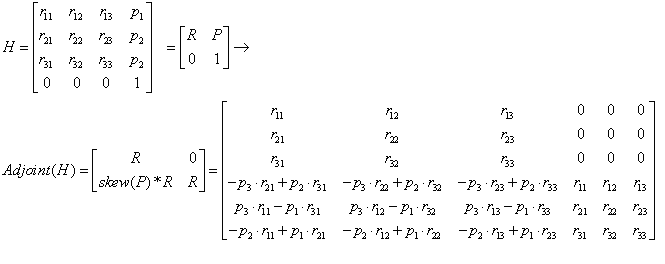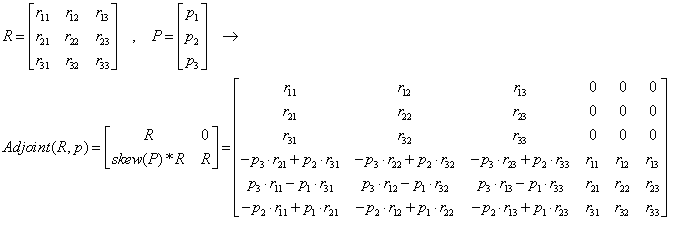﻿ 20-sim webhelp > Language Reference > Functions > Matrix > Adjoint

## Description

Returns the adjoint matrix [6,6] of a homogeneous matrix [4,4]:or returns the adjoint matrix [6,6] of a rotation matrix R [3,3] and position vector P :The Adjoint function is useful for dynamics described by twists and wrenches.

## Examples

R = [cos(alpha),-sin(alpha),0 ; sin(alpha),cos(alpha),0 ; 0,0,0];

P = [p1 ; p2 ; p3];